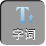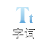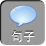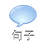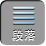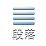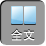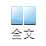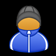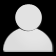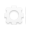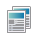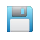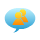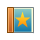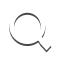-AA+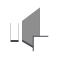18这八个自然数分别填入下图中的八个○内，使四边形每条边上的三个数之和都等于 14，且数字 1出现在四边形的一个顶点上.应如何填？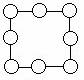【答案】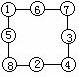【分析】 为了叙述方便，先在各圆圈内填上字母，如下图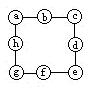a+ b+ c= 141

c+ d+ e= 142

e+ f+ g= 143

a+ h+ g= 144

a+ b+ c+ e+ f+ g= 28

a+ b+ c+ d+ e+ f+ g+ h-d+ h）= 28

d+ h=（ 1+ 2+ 3+ 4+ 5+ 6+ 7+ 8-28= 8

1,2,3,4,5,6,7,8中有 1+ 7= 2+ 6= 3+ 5= 8.

1要出现在顶点上， d+ hb+ f只能有 2+ 63+ 5两种填法；

aceg可取到 1478

a= 1，则 c= 14 -1+ 2）= 11，不在 1478中，不行；

c= 1，则 a= 14 -1+ 2）= 11，不行；

e= 1，则 c= 14 -1+ 3）= 10，不行；

g= 1，则 a= 8c= 4e= 7.